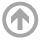Mathematics Department

# Combinatorics, Algebra, & Topology Seminar

## Spring 2021

• Mar
05
• Zero forcing and the ordered multiplicity Inverse eigenvalue sequence problem for graphs
Franklin Kenter
USNA
Time: 03:45 PM

#### View Abstract

Given a graph G, one may ask: What sets of eigenvalues are possible over all weighted adjacency matrices of G?'' (Here, negative and diagonal weights are allowed). This is known as the Inverse Eigenvalue Problem for graphs (IEPG) A mild relaxation of this question considers the multiplicity sequence instead of the exact eigenvalues themselves. For instance, given a graph G on n vertices and an ordered partition (m_1, ... , m_\ell) of n, is there a weighted adjacency matrix where the i-th distinct eigenvalue has multiplicity m_i? This is known as the ordered multiplicity IEPG. Recent work solved the ordered multiplicity IEPG for all graphs on 6 vertices. In this talk, we discuss the multiplicity IEPG and develop zero forcing methods for the ordered multiplicity IEPG in a multitude of different contexts. Namely, we utilize zero forcing parameters on powers of graphs to achieve bounds on consecutive multiplicities. Not only can we verify the above result in a more straight-forward manner, but we apply our techniques to skew-symmetric matrices and nonnegative matrices, among others.
• Feb
05
• Van Nguyen
USNA
Time: 03:45 PM

#### View Abstract

In a paper in 1962, Golod proved that the Betti sequence of the residue field of a local ring R attains an upper bound given by Serre if and only if the homology algebra A of the Koszul complex of R has trivial multiplications and trivial Massey operations. This is the origin of the notion of the Golod ring. In joint work with Oana Veliche, using the Koszul complex as building blocks, we construct a minimal free resolution for any local ring. We describe how the multiplicative structure and the triple Massey products of the homology algebra A are involved in the construction. As a result, we provide explicit formulas for the first six terms of a sequence that measures how far the ring R is from being Golod, and discuss other consequences of this construction. This talk is at Max's request and my first work in the commutative algebra area. Necessary background will be given.

#### ARCHIVES